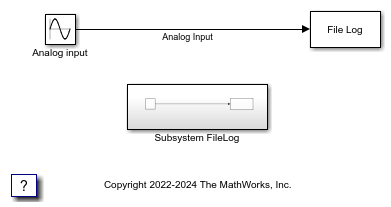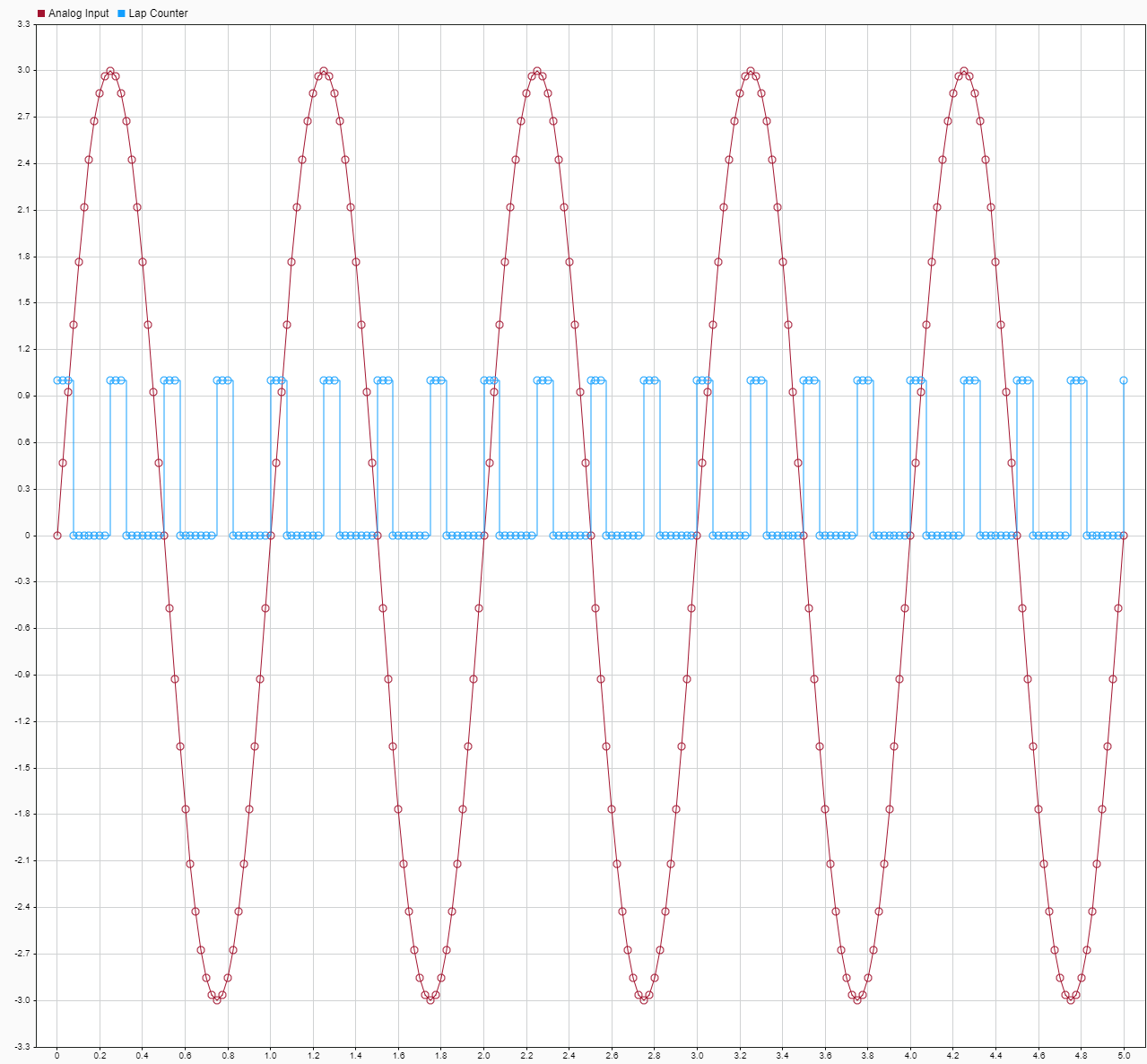# Tune Decimation for File Log Data Without Model Rebuild

This example shows how to tune the decimation parameter on the File Log blocks in a real-time application without rebuilding the model. The `Application` object methods `getAllFileLogBlocks`, `getFileLogDecimation`, and `setFileLogDecimation` are used to change the decimation value for the application MLDATX file. The application can be run again on the target computer to observe the updated decimation of the signals connected to File Log blocks in the model.

Open the model `slrt_ex_filelogtunabledecimation>`. This model uses File Log blocks to log data on the target computer. The default setting for decimation is set to 1 for the File Log blocks. In the MATLAB Command Window, type:

```model = 'slrt_ex_filelogtunabledecimation'; open_system(fullfile(matlabroot,'toolbox','slrealtime','examples',model)); ```• Configure for a non-Verbose build.

```set_param(model,'RTWVerbose','off'); set_param(model,'StopTime','5'); evalc('slbuild(model)'); tg = slrealtime; load(tg,model); ```

### Close the model

```bdclose(model); ```

### Run Real-Time Application

Run the real-time application on the target computer. Wait for the application to stop.

```start(tg); pause(7); ```

### View Signals in the Simulation Data Inspector

```Simulink.sdi.view; ```### Set File Log Block Decimation

Set the File Log block decimation for the blocks to the same value by using the `Application` object. Create the `Application` object.

```appObj = slrealtime.Application(model); ```

Get the File Log blocks in the application by using the `getAllFileLogBlocks` function.

```fileLogBlocks = appObj.getAllFileLogBlocks; ```

Get the File Log decimation setting for the blocks by using the `getFileLogDecimation` function. The setting is 1 for both blocks.

```oldDecimation = appObj.getFileLogDecimation(fileLogBlocks) ```
```oldDecimation = 1 ```

Change the decimation for both blocks to 5 by using the `setFileLogDecimation` function.

```appObj.setFileLogDecimation(fileLogBlocks, 5); ```

Confirm the new decimation value is set to 5.

```newDecimation = appObj.getFileLogDecimation(fileLogBlocks) ```
```newDecimation = 5 ```

### Reload Application on Target Computer and Rerun

```load(tg,model); start(tg); pause(7); ```

### View New Signals in the Simulation Data Inspector

```Simulink.sdi.view; ```### Set File Log Block Decimation

Set the File Log block decimation for blocks to different values by using the `Application` object. Create the `Application` object

```appObj = slrealtime.Application(model); ```

Get the File Log blocks in the application by using the `getAllFileLogBlocks` function.

```fileLogBlocks = appObj.getAllFileLogBlocks; ```

Get the File Log decimation setting for the blocks by using the `getFileLogDecimation` function. The setting is 1 for both blocks.

```oldDecimation = appObj.getFileLogDecimation(fileLogBlocks) ```
```oldDecimation = 5 ```

Change the decimation for both blocks to 5 by using the `setFileLogDecimation` function.

```appObj.setFileLogDecimation(fileLogBlocks, [1 2]); ```

Confirm the new decimation value is set to 5.

```newDecimation = appObj.getFileLogDecimation(fileLogBlocks) ```
```newDecimation = 1 2 ```

### Reload Real-Time Application and Rerun

```load(tg,model); start(tg); pause(7); ```

### View Signals in the Simulation Data Inspector

```Simulink.sdi.view; ```